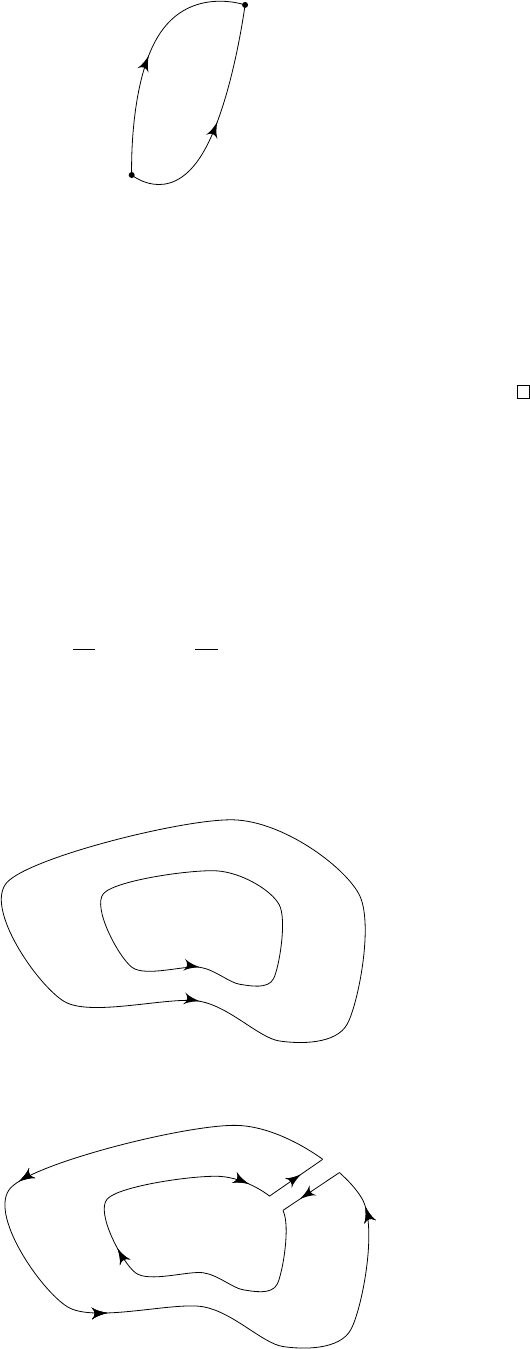2Contour integration and Cauchy's theorem

IB Complex Methods2.3 Contour deformation
One useful consequence of Cauchy’s theorem is that we can freely deform contours
along regions where f is defined without changing the value of the integral.
Proposition.
Suppose that
γ
1
and
γ
2
are contours from
a
to
b
, and that
f
is
analytic on the contours and between the contours. Then
Z
γ
1
f(z) dz =
Z
γ
2
f(z) dz.
a
b
γ
2
γ
1
Proof.
Suppose first that
γ
1
and
γ
2
do not cross. Then
γ
1
γ
2
is a simple closed
contour. So
I
γ
1
γ
2
f(z) dz = 0
by Cauchy’s theorem. Then the result follows.
If
γ
1
and
γ
2
do cross, then dissect them at each crossing point, and apply
the previous result to each section.
So we conclude that if
f
has no singularities, then
R
b
a
f
(
z
) d
z
does not depend
on the chosen contour.
This result of path independence, and indeed Cauchy’s theorem itself, becomes
less surprising if we think of
R
f(z) dz as a path integral in R
2
, because
f(z) dz = (u + iv)(dz + i dy) = (u + iv) dx + (v + iu) dy
is an exact differential, since
y
(u + iv) =
x
(v + iu)
from the Cauchy-Riemann equations.
The same idea of “moving the contour” applies to closed contours. Suppose
that
γ
1
is a closed contour that can be continuously deformed to another one,
γ
2
, inside it; and suppose f has no singularities in the region between them.
γ
2
γ
1
××
×
We can instead consider the following contour γ:
γ
××
×
By Cauchy’s theorem, we know
H
γ
f
(
z
) d
z
= 0 since
f
(
z
) is analytic throughout
the region enclosed by
γ
. Now we let the distance between the two “cross-cuts”
tend to zero: those contributions cancel and, in the limit, we have
I
γ
1
γ
2
f(z) dz = 0.
hence we know
I
γ
1
f(z) dz =
I
γ
2
f(z) dz = 0.